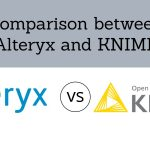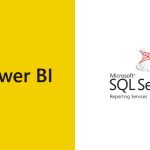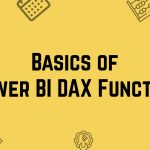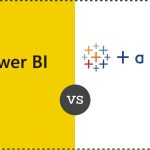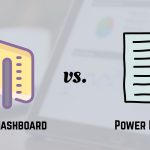[email protected] +1 203-349-9909 / +91-8080042523

Formulas

How to use Clean Function in Excel

The CLEAN Function in Excel takes a text string and returns a text that has been “cleaned” of line breaks and other non-printable characters. What is a CLEAN Function? What is the syntax for CLEAN Function? How to use CLEAN Function? And finally, What are the applications of CLEAN Function? ➡ What is Clean Function in...

How to use the Excel Choose Function

The CHOOSE Function in Excel returns a value from a list using a given position or index. The values provided to CHOOSE can include references. Let’s have a look below to get more details about CHOOSE Function. ➡ What is Excel Choose Function? CHOOSE Function is a function in Excel that helps you to get...

How to use the Excel Code Function

The CODE Function in Excel returns a numeric code for a given character. The CODE Function can be used to understand numeric codes given to the CHAR Function as it is the reverse process of CHAR Function. ➡ What is Code Function in Excel? The Function that accepts one argument, for the text for which you...

How to use the Excel Column Function

The COLUMN function in Excel returns the column number for a reference. In other words, Column Function too will return the column number of the cell which contains formula when reference is not provided. ➡ What is Column Function in Excel? The function which will returns column number for a reference is called Excel COLUMN Function....

How to use Ceiling Function in Excel

The CEILING Function in Excel returns a given number rounded up to a specified multiple. CEILING Function is always used for rounding up. Its purpose is to Round a number up to nearest multiple. It returns a rounded number. What is a CEILING Function in Excel? What is the syntax for CEILING Function? Where can...

How to use Char Function in Excel

The CHAR Function in Excel returns a character when given a valid Character Code. CHAR Function is used to specify the characters that are difficult to enter in a formula in Excel. Now, let us study in detail the CHAR Function as given below. What is a CHAR Function in Excel? What is the formula...

How to use AVERAGEIFS FUNCTION in Excel

The AVERAGEIFS Function of Excel computes the average of the figures in a range that meet one or more delivered conditions. The criteria of the AVERAGEIFS Function also supports the logical operators and wildcard operators in Excel. What Is The Averageifs Function In Excel? AVERAGEIFS Function averages cells in a range that is equivalent to...

How to use AVERAGEIF FUNCTION

The AVERAGEIF Function of Excel calculates the average of the numbers in a range that meet the supplied conditions. The measures for AVERAGEIF Function supports logical operators and wildcards for partial matching. What is an AVERAGEIF Function in Excel? What is the formula for AVERAGEIF Function? What is the example of AVERAGEIF Function? How is...

How to use ABS Function in Excel

An Excel Function is a predefined formula that executes the actions like calculating values in a precise order. The common functions of Excel are AGGREGATE, AVERAGE, COUNT, INDEX, ROUND, SUM etc. In this blog, we are going to see all the Excel Functions one by one in depth. Also, you will learn the different parts of a function and how to...

How to use AND Function in Excel

An Excel Function is a predefined formula that executes the actions like calculating values in a precise order. The common functions of Excel are AGGREGATE, AVERAGE, COUNT, INDEX, ROUND, SUM etc. In this blog, we are going to see all the Excel Functions one by one in depth. Also, you will learn the different parts of a function and how to...

OFFSET Function in Excel

OFFSET Function in Excel: Most Useful Built-in-Functions in Excel. It returns a range given to the no. of Rows and Columns. The User gives the Row, Column and Reference to a range and wherever the Row and Columns meet, the Offset Formula in Excel will give the Output based on that. What is an OFFSET...

INDIRECT Function with SUM, MAX, MIN & Independent Cell Value

What is an INDIRECT Function? The INDIRECT Function in Excel helps you to return a reference to a range. Even when you insert Rows or Columns in a Worksheet the reference you created won’t change. This will help you to create a reference from letters and numbers in other cells. How INDIRECT Function Works with an...

What is ISFORMULA Function and FORMULATEXT Function

In our tutorial today, we will be looking at some new functions in Excel 2013, these are ISFORMULA and FORMULATEXT Functions. Let’s take them one after the other. The ISFORMULA Function Gone are the days when it is difficult to identify cells that contain formulas. Some time ago, we have to create a user-defined function...

How to Use SUBSTITUTE Function

Let’s first grasp what a Substitute Function is and when to use it. Before we figure out how to use the SUBSTITUTE function. What is a Substitute function? A Substitute is a person or a thing acting or serving in place of another. Substitute function replaces/swaps a set of characters with another in a text string. For...

Quartile Function in Excel

Summary: The Quartile Function in Excel helps to return the quartile of a data set in Excel. Going further in this tutorial, you’ll see how to find Quartile using Excel. You’ll observe that the Quartiles are several times used in sales and also while inspecting the sample data. The Quartile Function in Excel will support you...

How to use the Excel PERCENTILE function

Excel PERCENTILE Function: Summary  The Percentile Function in Excel will return the k-th percentile of values in a range. This implies that the value below the k% of the data values fall, while using the Percentile Function. This is for a Supplied range of values also for the supplied k. The Percentile Function in Excel...

How to use TEXTJOIN, IFS, SWITCH, MAXIFS and MINIFS Functions?

I am pleased to introduce you to some of the New Excel Functions in Excel 2016. These functions are ‘wow!!’. The functions I will be introducing in this tutorial are TEXTJOIN, IFS, SWITCH, MAXIFS and MINIFS. Let’s take them one after the other. The TEXTJOIN Function As the name implies, this function is used to...

How to compare two lists in Excel

In the tutorial, we will learn: How to compare two columns in Excel. We use various ways to compare two cells using functions in Excel. Some of these ways are: Comparing two Columns row-by-row. Comparing many columns for row matches or differences. Highlight matches or differences between two columns. Highlight row matches and differences. Compare...

Extract values from cell using LEFT, RIGHT, MID

Imagine that you wanted to create a new column on a spreadsheet. But part of the information is already in another column? For example: You wanted to distribute the first, middle and last names from a cell into three separate cells. Do you bristle when you see someone wasting precious time for this? Case study...
1 2